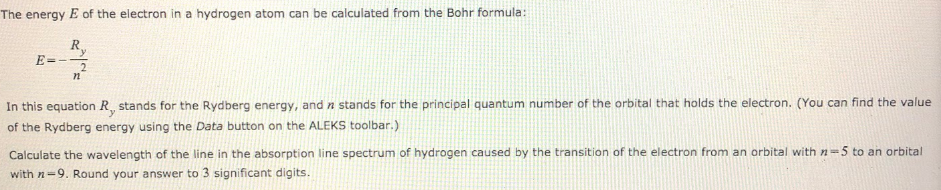# The energy E of the electron in a hydrogen atom can be calculated from Bohr formula: E=-Ry/n^2 In this equation Ry stands for the Rydberg energy, and n stands for the principal quantum number of the orbital that holds the electron. ( You can find the value of the Rydberg energy using the Data button on the ALEKS toolbar.) Calculate the wavelength of the line in the absorption line spectrum of hydrogen caused by the transition of the electron from an orbital with n = 5 to an orbital with n = 9. Round your answer to 3 significant digits.